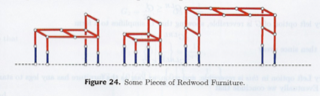# Monthly Archives: September 2019

## Left-Right Hackenbush for Redwood Furniture

Here’s an interesting problem, but a hard one to explain.  Most of what I’m doing here comes from the very cool “Winning Ways for Your Mathematical Plays” book by Berlekamp, Conway, and Guy, which I hope at some point in the future to have the time to really dig deeply into.  But for now, I’ll just use it as a reference to this week’s problem.

The problem: Left-Right Hackenbush for Redwood Furniture.  This is problem GP12 in the appendix.

The description: Ok, here we go.  First, a Hackenbush problem consists of an undirected, connected graph.  The edges of the graph are marked as “Left” or “Right”(though the book has some very nice colored pictures, where the edges are labeled “Blue” and “Red”).  Some of the vertices are on the ground (In G&J’s definition, there is one ground vertex, but it’s equivalent to having several vertices that are all on the ground).

On Left’s turn, they remove a blue edge and then all edges that are not connected to the ground are removed.  On Right’s turn, they remove a red edge, and then all edges that are not connected to the ground are removed.  A player loses if there are no remaining edges of their color.

redwood furniture Hackenbush instance is one where:

• No red edges touch the ground
• Each blue edge (or “foot”) has one end on the ground and the other touches a unique red edge (the “leg”)

Here are some redwood furniture instances from the book:The “value” of a Hackenbush position is the number of “spare” moves (with optimal play) one player has after the other player loses.  A value of 0 means that whoever’s turn it is will lose (on an empty board).  The definition can be extended to fractional values.  For example, a value of 1/2 for (say) Left means that if we made two copies of the game, we would end up with a situation with a value of 1 for Left.

So, the question is, for some Redwood Furniture graph, and some K, is the value <= 2-K?

Reduction:

I’m just going to sketch the process here since it takes several pages of the book (and depends on results and ideas from earlier in the book).

They show:

• The value of any redwood furniture graph is 2-N for some N.  In the degenerate case, the graph with just one Left edge has a value of 1. (=20)
• On Left’s turn, they will always remove a foot (by definition, that’s all they have).  On Right’s turn, they should make a move that does not disconnect the graph, if possible.
• A “Bed” is a redwood furniture graph where every red edge that is not a leg connects to a leg.  It has value 2-(m+1), where m is the largest number of moves that do not disconnect the bed.
• The value of the game depends on how many extra moves Red has to get down to just a bed.
• To find m, you need to know the size of the largest redwood tree (a tree is a graph that will be disconnected by the removal of any edge) that contains all of the legs.
• The edges of the bed (the red edges that are not legs) form a bipartite graph.  So finding m is equivalent to the set covering problem, where the elements of the set are the vertices, and the edges are the sets.

Here’s how I think the Set Covering reduction works.  Given a set covering instance: a set S of elements, and a collection C of subsets of S, we’ll build a bipartite graph.  One set of the bipartite graph will correspond to the elements of S (and will be on the legs of the furniture graph).  The other set will correspond to the elements in C (and will be the vertices in the bed that are not in the legs).  An edge will go from each “C vertex” to each “S vertex” in its set.  Now, the cover is the set of vertices from the bed that cover all of the legs.

The book says you want the “smallest redwood tree which contains all of the legs”, which I think is the same thing (smallest number of extra vertices), but I’m not 100% confident since the Hackenbush game involves removing edges, and we’re choosing vertices in the cover.

I’m a little sad that the book does such a great job describing the game, and the value function, and then glosses over the reduction part (and uses misleading terms like “Minimum Spanning Tree of a Bipartite Graph”, which is a polynomial problem).  The actual reduction in G&J is to a private communication, so that’s not much help.

Difficulty: Boy, I don’t know, I think it depends on where you start from.  If my Set Cover reduction is the right one, and all you ask a student to do is that, it’s probably a 4.  If you’re going to make them prove all of the things I glossed over about the value number, then it probably goes up to at least an 8.